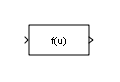Documentation

### This is machine translation

Mouseover text to see original. Click the button below to return to the English version of the page.

To view all translated materials including this page, select Country from the country navigator on the bottom of this page.

# Fcn

Apply specified expression to input

• Library:
• Simulink / User-Defined Functions## Description

The Fcn block applies the specified mathematical expression to its input. The expression can include one or more of these components:

• `u` — The input to the block. If `u` is a vector, `u(i)` represents the `i`th element of the vector; `u(1)` or `u` alone represents the first element.

• Numeric constants.

• Arithmetic operators (+ - `*` / ^).

• Relational operators (`== != > < >= <=`) — The expression returns 1 if the relation is true; otherwise, it returns 0.

• Logical operators (&& || !) — The expression returns 1 if the relation is true; otherwise, it returns 0.

• Parentheses.

• Mathematical functions — `abs`, `acos`, `asin`, `atan`, `atan2`, `ceil`, `cos`, `cosh`, `exp`, `floor`, `hypot`, `log`, `log10`, `power`, `rem`, `sgn` (equivalent to `sign` in MATLAB®), `sin`, `sinh`, `sqrt`, `tan`, and `tanh`.

### Note

The Fcn block does not support `round` and `fix`. Use the Rounding Function block to apply these rounding modes.

• Workspace variables — Variable names that are not recognized in the preceding list of items are passed to MATLAB for evaluation. Matrix or vector elements must be specifically referenced (e.g., `A(1,1)` instead of `A` for the first element in the matrix).

The Fcn block observes the following rules of operator precedence:

1. `( )`

2. `^`

3. `+ - `(unary)

4. `!`

5. `* /`

6. `+ -`

7. `> < <= >=`

8. `== !=`

9. `&&`

10. `||`

The expression differs from a MATLAB expression in that the expression cannot perform matrix computations. Also, this block does not support the colon operator (:).

Block input can be a scalar or vector. The output is always a scalar. For vector output, consider using the Math Function block. If a block input is a vector and the function operates on input elements individually (for example, the `sin` function), the block operates on only the first vector element.

## Ports

### Input

expand all

The Fcn block accepts and outputs signals of type `single` or `double`.

Data Types: `single` | `double`

### Output

expand all

The Fcn block accepts and outputs signals of type `single` or `double`.

Data Types: `single` | `double`

## Parameters

expand all

Specify the mathematical expression to apply to the input. Expression components are listed above. The expression must be mathematically well-formed (uses matched parentheses, proper number of function arguments, and so on). The expression has restrictions on tunability (see Limitations).

#### Programmatic Use

 Block Parameter: `Expr` Type: character vector Value: mathematical expression Default: `'sin(u(1)*exp(2.3*(-u(2))))'`

### Note

This parameter is not visible in the block dialog box unless it is explicitly set to a value other than `-1`. To learn more, see Blocks for Which Sample Time Is Not Recommended.

## Block Characteristics

 Data Types `double` | `single` Direct Feedthrough `No` Multidimensional Signals `No` Variable-Size Signals `No` Zero-Crossing Detection `No`